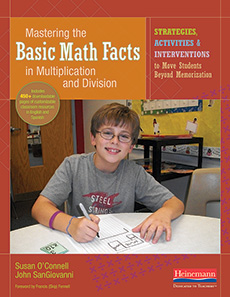# Thanks for your interest in Mastering the Basic Math Facts: Multiplication and Division!Helping students learn their math facts in multiplication and division can be challenging. This book from Susan O’Connell and John SanGiovanni focuses on why math facts are crucial and how to help students master them in ways that stick and lead to greater mathematical understanding and flexibility.

In this sample chapter, you’ll find:

• An overview of why basic math facts are so important and what constitutes “mastery”
• A look at the kind of learning that leads to conceptual understanding and strategic thinking
• Practical ideas and activities to try from the chapter focused on multiplying by 5